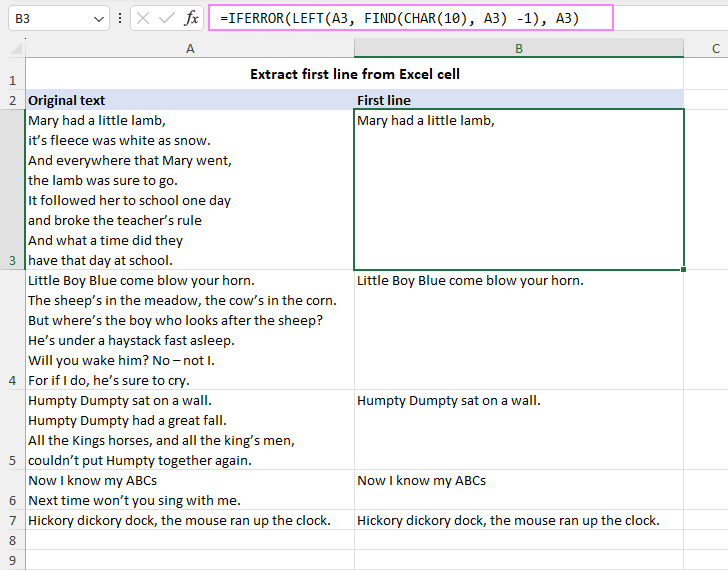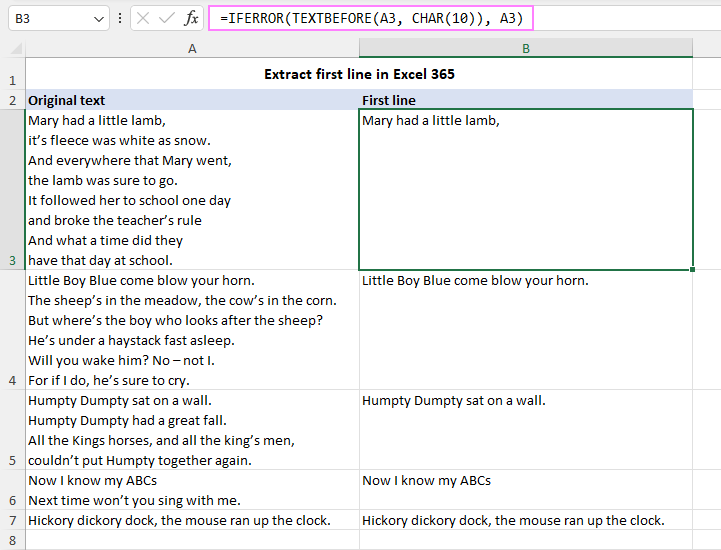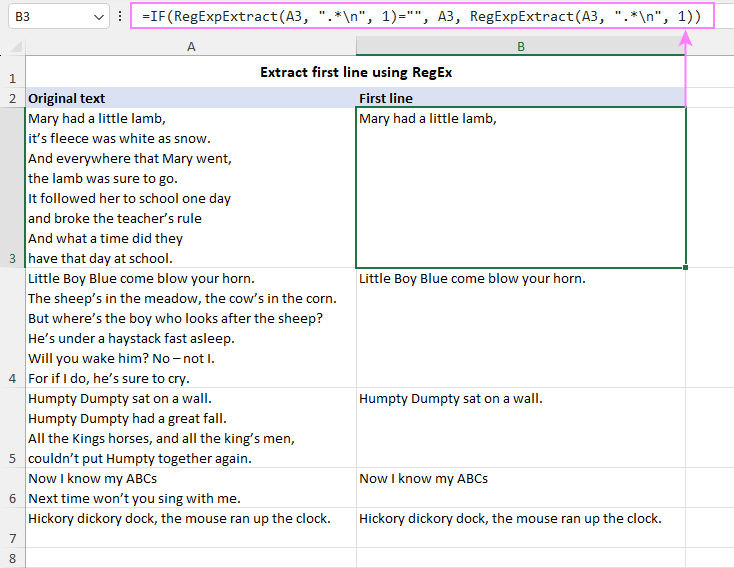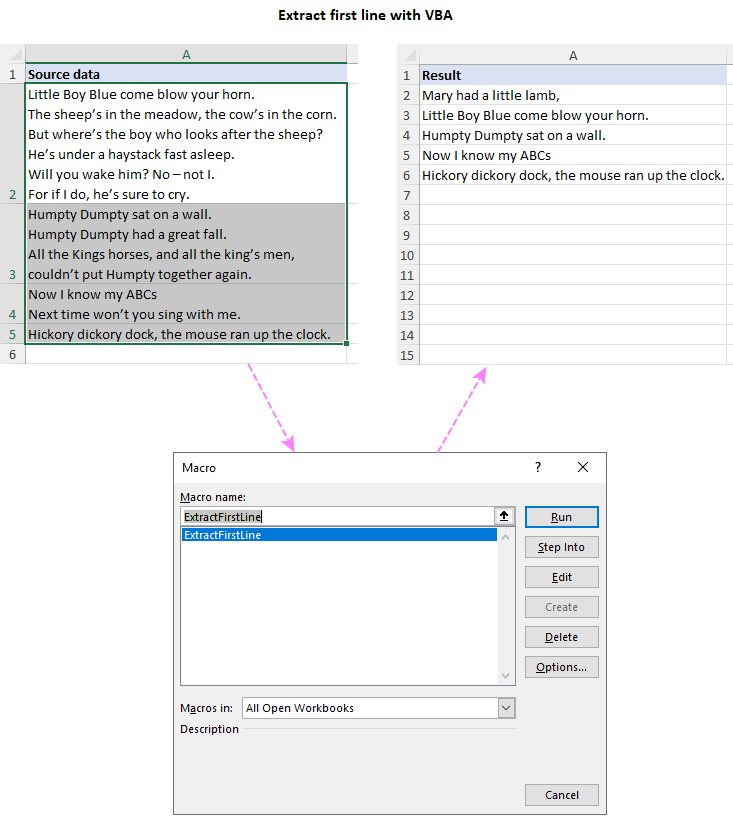# How to extract first line in Excel cell

In this tutorial, you will learn 3 effective approaches to extracting the first line of text from Excel cells: formulas, regex, and VBA macros.

In the world of data analysis, Excel stands as a powerful tool that offers various features to optimize complex tasks. One such task is extracting specific content from a cell, a technique that can prove invaluable in presenting data efficiently. This article delves into various methods of extracting the first line from a multi-line cell, discussing both formula-based approaches and VBA macro.

## How to extract the first line in Excel cell with formula

Formulas in Excel are the backbone of data manipulation. When it comes to extracting the first line from a cell, the following steps outline a straightforward method for achieving this:

1. Identify the line break within the text. This can be done with the help of the CHAR(10) function.
2. Locate the position of the new line character. To pinpoint the exact position of the first line break within the cell's content, the FIND or SEARCH function can be employed. The formula variations for these functions are as follows:

`FIND(CHAR(10), cell)` or `SEARCH(CHAR(10), cell)`

3. Adjust for position. As Excel counts positions from 1, while programming languages usually count from 0, subtract 1 from the line break position. This adjustment ensures accurate text extraction without including the carriage return character itself:

`FIND(CHAR(10), cell) -1`

4. Extract text before the line break. With the position of the line break known, you can now use the LEFT function to extract the text up to that point.

The generic formula takes this form:

LEFT(cell, FIND(CHAR(10), cell) -1)

For instance, if the original text is stored in cell A3, the formula would appear as:

`=LEFT(A3, FIND(CHAR(10), A3) -1)`

The formula works beautifully for multi-line cells. However, a noteworthy caveat arises when dealing with single-line cells. In such cases, the formula returns the #VALUE error because the FIND function fails to locate the carriage return character CHAR(10) within the cell.

To address this limitation, an elegant solution involves encasing the core formula within the IFERROR function:

`=IFERROR(LEFT(A3, FIND(CHAR(10), A3) -1), A3)`

This refined formula preserves the original cell content in cases where no line break is detected, effectively eliminating the error.By learning these nuances, you can confidently use formulas to grab the first line from Excel cells, no matter the situation.

## Get the first line of text in Excel 365

In Excel 365, getting the first line from a cell can be accomplished efficiently using the TEXTBEFORE function, which allows you to extract text before a specified character. In this case, the character we're interested in is the newline character, represented by CHAR(10).

TEXTBEFORE(cell, CHAR(10))

Assuming the original text is stored in cell A3, the formula to extract the first line would look like this:

`=TEXTBEFORE(A3, CHAR(10))`

To ensure a smooth experience when dealing with cells containing only a single line of text, wrap the core formula with the IFERROR function. This approach maintains the original cell content in instances where a line break is absent, preventing any error messages.

`=IFERROR(TEXTBEFORE(A3, CHAR(10)), A3)`

This formula-driven technique guarantees a reliable method for extracting the first line of text from Excel 365 cells, irrespective of whether they contain multiple lines or just one line.## Keep only the first line of text using Excel Regex

Another solution to only keep the first line and discard the rest is by using regular expressions. This method introduces a custom function named RegExpExtract, which follows this syntax:

RegExpExtract(text, pattern, [instance_num], [match_case])

The idea behind RegExpExtract is to use a regular expression pattern that matches everything before the new line character (\n) in the original cell. Then, you can pull that part of the text into another cell by using this formula:

RegExpExtract(cell, ".*\n", 1)

Breaking down the components of this generic formula:

• Cell - is the reference to the cell that contains multiple lines of text.
• ".*\n" - is the regex pattern that matches any character (.) repeated zero or more times (*) followed by a new line character (\n).
• 1 - is the instance number, specifying that we want to extract only the first occurrence of the pattern in the cell.

For example, to copy the first line of text from cell A3 into cell B3, utilize this simple formula:

`=RegExpExtract(A3, ".*\n", 1)`

However, there is one caveat with it. If the source cell only contains one line of text, without any new line character, then the formula will return nothing (empty string). This is because there is no match for the pattern ".*\n" in such cases.

To fix this issue, you can use an IF statement that checks if there is any match for the pattern in the cell. If there is no match, then you simply return the original cell value. Otherwise, you extract the first match as before. The modified formula looks like this:

`=IF(RegExpExtract(A3, ".*\n", 1)="", A3, RegExpExtract(A3, ".*\n", 1))`

This improved version of the formula ensures that you always get some value in column B, whether it is one line or multiple lines of text in column A.To use this formula in your own workbook, you need to add the VBA code for the RegExpExtract function first. You can find the code in this article: Excel VBA Regex function to extract strings. Alternatively, you can download the sample workbook at the end of this tutorial and copy the code from there. Once you have the code in your workbook, you can use the RegExpExtract function just like any native Excel function.

Tip. If you are looking for an easier and faster way to extract text strings in Excel using regular expressions, then check out Regex Tools included with our Ultimate Suite for Excel. Integrated seamlessly in Excel, the tools can perform a variety of text string operations. No VBA code required.

## Copy the first line of text to another sheet with Excel VBA

To automate the process of extracting the first lines from cells within a selected range and relocating them to a new worksheet, a VBA macro presents an effective solution. This section outlines the macro, providing step-by-step instructions on how to implement it and utilize its capabilities.

How to use the macro:

1. Add the code. Integrate the provided code into your workbook following the guidelines in How to insert VBA code in Excel.
2. Select the range. Choose the range of cells containing the text you want to extract the first line from.
3. Run the macro. Press Alt + F8 to open the Macro dialog box. Select the ExtractFirstLine macro and click Run to execute the code.

As a result, the macro will create a new sheet named "Extract 1st line - results" and copy the extracted first lines from the selected range into that sheet.

Important points to note:

1. If no range is selected, the macro prompts you to select a range, which can include just a single cell.
2. The macro utilizes Chr(10) as the delimiter to extract the first line of text. If needed, you can specify a different delimiter in the code to get text before it.
3. Empty cells are ignored during extraction.
4. If a cell is not empty but the specified delimiter is not found, the entire content of the cell is returned.
5. If you want the resulting worksheet to be named differently, you can either rename the sheet tab directly in Excel or specify the desired sheet name within the VBA code.

By utilizing this Excel VBA macro, you can swiftly extract the first lines of text from cells and organize them in a new worksheet. You can also adjust the macro to your preferences, such as using a different delimiter or changing the name of the result sheet.In essence, mastering the art of getting the first line from an Excel cell is like blending your tech wizardry with creative ingenuity. Whether you opt for the precision of formulas, the versatility of regex, or the automation of VBA macros, there's more than one road to the same result. Throughout this guide, we've sliced, diced, and compared these three tactics, dishing out some handy tips along the way. I hope you enjoyed this tutorial and can now dive into your next data adventure armed with new knowledge 😊